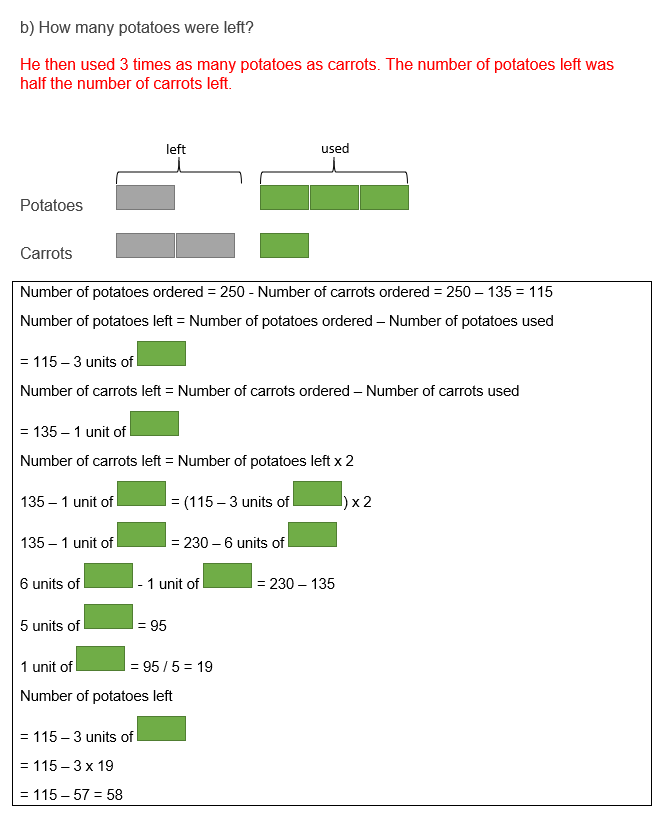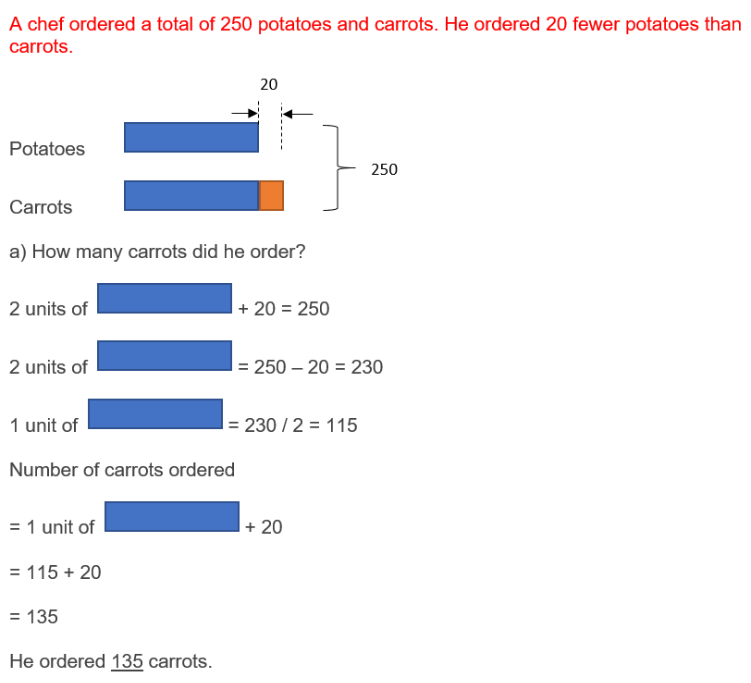# Question

A chef ordered a total of 250 potatoes and carrots. He ordered 20 fewer potatoes than carrots. He then used 3 times as many potatoes as carrots. The number of potatoes left was half the number of carrots left.

a) How many carrots did he order?

b) How many potatoes were left?

potatoes —
left : 1u
used : 3p

carrots —
left : 2u
used : 1p

250 – 20 = 230
230/2 = 115 (potatoes) ——- 1u + 3p
230 ——- 2u + 6p
115 + 20 = 135 (carrots) ——- 2u + 1p
1p ——- (230 – 135)/(6 – 1) = 19
1u ——- (135 – 19)/2 = 58 (b)
2u + 1p ——- 2 x 58 + 19 = 135 (a)

Ans : (a) 135 carrots; (b) 58 potatoes left.

0 Replies 0 Likes0 Replies 0 Likes0 Replies 0 Likes

This is a Before After question.

 Before Transfer After Potato 1u – 20   = 115 – 3a 115 – 3a Carrot 1u          = 135 – 1a 135 – 1a Total 2u – 20

a)

2u – 20 = 250

2u = 250 + 20 = 270

1u = 270 ÷ 2 = 135 carrot

b)

(115 – 3a ) / 1   = (135 – 1a) / 2

230 – 6a =  135 – 1a

230 – 135 = 6a – 1a

5a = 95

1a = 95 ÷ 5 = 19

3a = 19 × 3 =57

115 – 57 = 58 potato

0 Replies 0 Likes